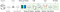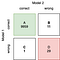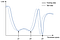# Why model validation and interpreting estimates is not trivial

## Investigating on issues leading to overestimated machine learning models

Part 4 of the article Validation of Machine Learning Models Focusing on Reliability

This article examines issues that might arise during model validation and final performance estimation leading to unreliable results. Amongst others, it addresses the following questions:

• Why the distinction between validation and performance estimation is crucial.
• How the test set can be turned into a training set accidentally and how to avoid it.
• Why a performance score might not be as significant as expected.

Given a properly prepared data set, the remaining tasks of the machine learning engineer can be combined into two:

1. Creating the best performing model including training, validation and optimisation as well as the selection of the final model out of potential candidates.
2. Providing an estimate of the model’s performance on future data


Those steps are highlighted in figure 1 showing a possible development flow of a model.Figure 1: The steps from training to the selection of the final model

Note that the terms validation and estimation are used in different ways. Model validation refers to the evaluation of the model as part of the optimisation process. The final performance estimation is done afterwards. This distinction directly indicates that there is room for errors. The next paragraph explains why it is so important to understand the difference.

# Optimisation and estimation on the same data set

The performance of a model partially depends on the configuration of the hyperparameters, such as learning rate, batch size and the number of layers. Those parameters are optimised by training and validating the model several times with different parameter settings. The problem is that they are fitted to the particular validation set and information is leaked into the model. The validation error eventually becomes a training error, which is not suitable to estimate the model’s generalisability.
Obviously the model itself is trained on a training set. However, parameter optimisation can be seen as a separate learning procedure which renders the test error less meaningful. If this so called apparent error is presented as a performance estimate of the model, it will presumably be overoptimistic. For a proper performance estimate, a separate test set is needed that is exclusively utilised for calculating the final test error. As soon as any adjustment based on the test result is made to the model, the test set becomes a training set again.
In other words, the model is overfitted to the holdout set. [2 ,p.102–103] [3 p.17] [11, p. 22] 

## The reusable holdout

A very interesting solution to this problem called “Thresholdout” has been proposed by C. Dwork et al. . The idea comes from differential privacy, which is a mechanism to protect data from attackers by making it unidentifiable. The basic concept is to restrict the access to the holdout set so it can only be examined indirectly through the thresholdout.

As shown in figure 2.1 it takes the training set T, the holdout set H and a function like for example the error or the accuracy of the model as input. The thresholdout outputs an estimate by comparing the average values of f(T) and f(H). If the difference between those values is below the sum of a certain threshold τ and some noise η, the value of f(T) is returned. Otherwise f(H)+ξ is returned as an estimate, where η and ξ are random variables from a Laplace distribution.

## Nested cross-validation

One approach is to use a validation set for optimisation and a separate test set for performance estimation. This does not require much computational power and is easy to do. For small data sets it is not always feasible though. The smaller the data set, the less stable the model is, meaning that it is very sensitive to changes in the training data. [11, p.30]
As already discussed in the section “Negative effects of data preparation on model performance estimation”, performing validation on a single data set is rather insecure, especially when data is scarce.

When a model is validated on a single validation set, the estimate of generalisation refers to this particular data fold. If the model has a high variance, a point estimate like that might be misleading since the performance of such models differs greatly depending on the data set.

This creates the need of two nested cross-validation procedures: One inner loop used for hyperparameter tuning, wrapped inside an outer cross-validation to calculate a representative performance estimate. [7, p. 5] [3, p. 17]

Figure 2.2 visualises the basic concept of nested cross-validation. Given a data set D, the procedure can be divided into the following steps.

1. The whole data set D is divided into k disjoint parts dᵢ for i = 1…k.
2. For each of the k parts a training set Tᵢ=D−dᵢ is created. In the example depicted in figure 2.1 k is 3.
3. The resulting subsets Tᵢ are again divided into j parts tᵢⱼ. This is where the inner cross-validation starts.
4. Given a set of parameters p the model is trained on the data set Tᵢ−tⱼ and validated on tⱼfor each parameter in p.
5. According to cross-validation the average score sᵢ is computed. Based on that score, the optimal parameter pᵢ is chosen and used to train the model Mₖ on the whole set Tᵢ.
The model is then propagated to the outer loop. Note that sᵢ is not representative for the true performance of the model.
6. The k models that originate from hyperparameter optimisation are now tested on the corresponding test set dᵢ. The resulting scores are statistically valid in regard to the fact that the test data was held back from the optimisation algorithm.
7. The averaged score is representative for a final performance estimate.
[5, p.323- 325] [3, p. 17–18]

Let’s have a look at the outcome of this procedure.
On the one hand nested cross-validation leaves us with k individual models, possibly trained with different hyperparameters.
On the other hand we obtain a test score for each model from which we can calculate the mean and variance.

One might ask the question “Which of those models do I choose?” . The answer is simple: You do not choose any of them.
This is very important since selecting the model with the highest accuracy would yield an overoptimistic result. Instead of the best parameters derived from a particular data set we are looking for generalisability.
In fact, the purpose of nested cross validation is not selecting the best model but obtaining a realistic estimation of the model that is going to be trained on the whole data set. [7, p. 4–5]

Nevertheless the results can provide information about which parameter is likely to produce the best model. If the estimates of the outer cross-validation have little variance, this indicates that the final model is likely to perform according to the averaged estimate.

# Overrating test results

Estimating the true performance of a model is challenging and considering a reasonable estimate to be the truth, can be tempting. However, attributing too much importance to a single test result is short-sighted.

Imagine you had trained two different classifiers A and B on the same training set and tested them on the same test set. According to the test data, model A has an accuracy of 87% while model B reaches 90%. How reasonable would it be to select the algorithm of B? How do you know whether the difference between the two performance estimates is real or just due to statistical variation?[6,7]
Assume the scores are normally distributed random variables. If the test was carried out several times, the scores would scatter around an average, some being a little lower, some a little higher. In our example, the question is: Do the accuracy values of A and B come from the same distribution or not? Or to put it differently: Do they differ due to statistical chance or are they significantly different? A statistical test can be used to address this issue.

## Hypothesis tests

A statistical test quantifies the likelihood to observe two samples given that they have the same distribution. Thus a rejection of the null hypothesis means that the samples are likely to come from different distributions which would indicate a significant difference between the model skills. If the null hypothesis was not rejected, the samples are likely to differ due to fluctuations. 
There are different types of significance tests and the selection should be done carefully.

Overrating test results since statistically invalidity creeps in quickly if the tests are not applied correctly, making the results useless. The paper of Thomas Dietterich (1998) provides a detailed comparison of hypothesis tests for comparing classifiers. He recommends to either apply the McNemar’s test or the 5x2 CV test depending on the runtime of the model.
The McNemar’s test is based on the contingency table which counts the incorrect and correct statements of the two models in comparison as shown in figure 3.1.Figure 3.1: Contingency table based on the example from Raschka (2018) [11, p. 36]

The statistic of the test is calculated as follows:

Assume a significance threshold of α=0.05 for the given example. The statistic χ2 would be 8.3.
Looking up that value in the Chi-squared distribution, we obtain a p-value of 0.0039, which is below the significance level. This leads to the rejection of the null hypothesis which means that the model performances are significantly different.[11, p. 36]
The goal of the test is to check whether the disagreements of the two classifiers differ significantly or not. Since the test is applied on a single test set, it requires a test set that is representative of the population and models having as little instability as possible.

## Robustness

When a model is very sensitive to disturbances in the input data, this is a sign of fragility or in other words a lack of robustness. Since perturbations are part of the reality a model is exposed to at some point, a model should not only reach a high accuracy score but also preserve correctness in case of perturbations. In particular models with a high-dimensional input space like image classifiers are likely to be fragile.

A popular example of such perturbations are adversarial examples. The images are added with noise, which is not noticeable to the human eye. In spite of the fact that the modified images nearly look like the original ones to humans, the predictions of the models can deteriorate extremely.[12, p. 2] Figure 3.2 shows an example. After adding adversarial noise to an image of a pig, the classifier detects an airliner instead of a pig.

The robustness of a model against such an attack is called adversarial robustness. [9,p.7]
It can be tested by quantifying the impact of such noise on the correctness of a model in different ways, for example by indicating the minimum change of an input that results in a failure. [9, p.19] An approach to enable robustness would be the minimisation of the loss resulting from a worst-case adversarial attack, which can be seen as a kind of stress test. [12, p. 2]

Take a look at the loss landscape
The loss landscape is a graphical representation of a model’s loss.
Figure 3.3 depicts the loss landscape of a fictional model. In reality the parameter space would be high dimensional, however this representation is sufficient for the purpose of understanding the concept.

There are three local minima determined by the parameters p1, p2 and p3. The models resulting from p1 and p2 produce nearly the same error on the training data even though their parameters do not resemble. According to L. Breiman (2001), this behaviour is called the multiplicity of models.
Two models can be totally different in terms of their parameters and still perform equally well on a given data set which implies that there can be many solutions to a problem. In such cases it is difficult to tell which model to choose. Breiman compares this phenomenon to people reporting the same facts and yet telling different stories. [15, p.206]Figure 3.3: Loss landscape of a model with multiple local minima

From the depiction of the loss landscape one could conclude that the third model was suited best since it has the lowest loss of the three.
Imagine this model was exposed to some slightly perturbed test data. The perturbation results in a shift of the loss land-scape, represented by the dashed curve. In this case model 3 would perform much worse than model 1 and 2 since its minimum is very sharp and a horizontal shift of the curve causes a large increase in the loss.
Model 1 and 2 however, would be less affected by the perturbations. In fact, a flatter minimum is less sensitive to variations of the model parameters or to put it differently it is more stable. Consequently flat minima show better generalisation than sharper ones. 

However, Huang et al. note that due to the exponential larger volume of flat minima in higher dimensional space, they are more likely to be found. The other way around, it is more difficult to reach a sharp minimum. Huang et al. call this the “Blessing of dimensionality”. 

# Summary

This article highlighted some difficulties when validating machine learning models and pointed out how challenging the assessment of performance estimates can be. Apart from accuracy and error scores, there are many more properties that can be analysed in terms of performance such as robustness and stability. Note that this article is only intended to give an impression of how little is actually covered with a naive validation approach.
To sum up, here are the main conclusions.

• Practice the separation of hyperparameter optimisation and performance estimation.
• One approach to apply hyperparameter optimisation is nested cross-validation.
• Use an entirely unused test set for final performance estimation.
• A single performance estimate does not necessarily imply truth and might be optimistic or pessimistic.
• Consider to check for significance of the difference between two models during comparison.
• Consider the fragility and robustness of a model in terms of data perturbations.
• Keep in mind that a model with a higher score does not necessarily perform better in reality.

After all interpretation of validation results should be handled with great care. Always preserve a critical eye on performance estimates and question whether the methods used are statistically valid.
On top of a proper validation and estimation procedure, it could be profitable to incorporate methods like stress testing, in order to get a bigger picture of the actual performance.

# References

 M. Last. “The uncertainty principle of cross-validation”. In: 2006 IEEE Inter-national Conference on Granular Computing. 2006 IEEE International Conference on Granular Computing. Atlanta, GA, USA: IEEE, 2006, pp. 275–280.isbn: 978–1–4244–0134–5. doi: 10.1109/GRC.2006.1635796. URL: http://ieeexplore.ieee.org/document/1635796/ (Accessed: 05/04/2020)

 Marcel Neunhoeffer and Sebastian Sternberg. “How Cross-Validation Can GoWrong and What to Do About It”. In:Political Analysis27.1 (Jan. 2019). Pub-lisher: Cambridge University Press, pp. 101–106.issn: 1047–1987, 1476–4989.doi:10.1017/pan.2018.39. URL: https://www.cambridge.org/core/journals/political-analysis/article/how-crossvalidation-can-go-wrong-and-what-to-do-about-it/CA8C4B470E27C99892AB978CE0A3AE29 (Accessed: 05/04/2020).

 RapidMiner.How to Correctly Validate Machine Learning Models. RapidMiner.Library Catalog: rapidminer.com Section: Whitepaper. URL: https://rapidminer.com/resource/correct-model-validation/ (Accessed: 04/16/2020)

 Cynthia Dwork et al. “The reusable holdout: Preserving validity in adaptive data analysis”. In:Science349.6248 (Aug. 7, 2015). Publisher: American Associa-tion for the Advancement of Science Section: Report, pp. 636–638.issn: 0036–8075, 1095–9203.doi:10.1126/science.aaa9375. URL: https://science.sciencemag.org/content/349/6248/636 (Accessed: 04/16/2020)

 Steven L. Salzberg. “On Comparing Classifiers: Pitfalls to Avoid and a Recom-mended Approach”. In:Data Mining and Knowledge Discovery1.3 (Sept. 1,1997), pp. 317–328.issn: 1573–756X.doi:10.1023/A:1009752403260. URL: https://doi.org/10.1023/A:1009752403260 (Accessed: 05/06/2020)

 Jason Brownlee.Statistical Significance Tests for Comparing Machine Learn-ing Algorithms. Machine Learning Mastery. Library Catalog: machinelearning-mastery.com. June 19, 2018.url:https : / / machinelearningmastery .com/statistical- significance- tests- for- comparing- machine-learning-algorithms/(Accessed: 05/07/2020).

 Zoltan Prekopcsak, Tamas Henk, and Csaba Gaspar-Papanek.Cross-validation: The illusion of reliable performance estimation. URL: http://prekopcsak.hu/papers/preko-2010-rcomm.pdf (Accessed: 05/04/2020).

 Thomas G. Dietterich. “Approximate Statistical Tests for Comparing SupervisedClassification Learning Algorithms”. In:Neural Computation10.7 (Oct. 1998),pp. 1895–1923.issn: 0899–7667, 1530–888X.doi:10.1162/089976698300017197. URL: http://www.mitpressjournals.org/doi/10.1162/089976698300017197 (Accessed: 07/25/2020)

 ie M. Zhang et al. “Machine Learning Testing: Survey, Landscapes and Hori-zons”. In:arXiv:1906.10742 [cs, stat](Dec. 21, 2019). arXiv:1906.10742. URL: http://arxiv.org/abs/1906.10742 (Accessed: 05/04/2020)

 W. Ronny Huang et al. “Understanding Generalization through Visualizations”.In:arXiv:1906.03291 [cs, stat](July 16, 2019). arXiv:1906.03291 URL: http://arxiv.org/abs/1906.03291 (Accessed: 05/14/2020).

 Sebastian Raschka. “Model Evaluation, Model Selection, and Algorithm Se-lection in Machine Learning”. In:arXiv:1811.12808 [cs, stat](Dec. 2, 2018).arXiv:1811.12808. URL: http://arxiv.org/abs/1811.12808 (Accessed:05/04/2020).

 Suchi Saria and Adarsh Subbaswamy. “Tutorial: Safe and Reliable MachineLearning”. In:arXiv:1904.07204 [cs](Apr. 15, 2019). arXiv:1904 . 07204. URL: http://arxiv.org/abs/1904.07204 (Accessed: 04/20/2020)

Software Developer@Uxma | #programming #data science #web || #philosophy #ethics #society

## More from Jule M

Software Developer@Uxma | #programming #data science #web || #philosophy #ethics #society